# Climate Science Glossary

## Term Lookup

Enter a term in the search box to find its definition.

## Settings

Use the controls in the far right panel to increase or decrease the number of terms automatically displayed (or to completely turn that feature off).

# Settings

All IPCC definitions taken from Climate Change 2007: The Physical Science Basis. Working Group I Contribution to the Fourth Assessment Report of the Intergovernmental Panel on Climate Change, Annex I, Glossary, pp. 941-954. Cambridge University Press.HomeArgumentsSoftwareResources CommentsThe Consensus ProjectTranslationsAbout Support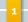Climate's changed before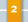It's the sun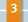It's not bad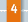There is no consensus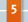It's cooling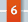Models are unreliable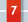Temp record is unreliable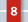Animals and plants can adapt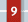It hasn't warmed since 1998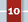Antarctica is gaining ice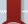View All Arguments...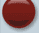## Latest Posts

Archives## OA not OK part 8: 170 to 1

#### Posted on 21 July 2011 by Doug Mackie

This is post 8 in a series about ocean acidification. Other posts: Introduction , 1, 2, 3, 4, 5, 6, 7, 8, 9, 10, 11, 12, 13, 14, 15, 16, 17, 18, Summary 1 of 2, Summary 2 of 2.

Welcome to the 8th post in our series about the fundamental chemistry of ocean acidification. In this post we show that adding acid to seawater removes carbonate.

In the last post we introduced the concept of reaction coefficient to describe the state of a reaction relative to the equilibrium constant. For equation 8 we have the following expressions: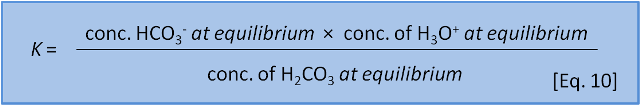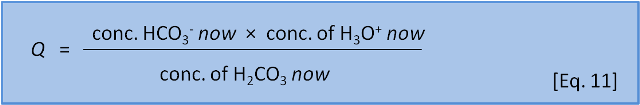The direction in which a reaction responds to a disturbance is given by comparing Q to K. If Q < K then the reaction system is not yet at equilibrium and favours the right side (products) to reach equilibrium. If Q > K then the reaction system has 'gone past' equilibrium and favours the left side (reactants) to return equilibrium. (If Q = K the system is at equilibrium). K values cover a wide range and pK, analogous to pH, is more commonly used: pK = -log(K) and K = 10-pK.

Consider Equation 8 at equilibrium (i.e. Q = K) and ask what happens if we add some acid H3O+ to the system. By adding H3O+ we increase the products, thus increasing the numerator and therefore increasing Q so that now Q > K. The reaction system therefore favours the left side (reactants) and H2CO3 is produced.

So far this sounds like Le Chatelier, but now we consider what happens with equation 8 and equation 9 occurring simultaneously.

For Eq. 8, in seawater at 10 oC, pK = 6.00. At the preindustrial ocean pH of 8.25, the equilibrium ratio of the left side, carbonic acid, to the right side, bicarbonate, is about 1:170 (depending on salinity). The calculation is 10-6.00 / 10-8.25. For Eq. 9, in seawater at 10 oC, pK = 9.19. At the preindustrial ocean pH, the equilibrium ratio of the left side, bicarbonate, to the right side, carbonate, is about 9:1 (the calculation is 10-9.19/10-8.25).If we couple these reactions, we see that at typical ocean pH equation 8 produces a ratio of carbonic acid to bicarbonate (and carbonic acid to H3O+) of 1:170. If we do add a little acid (H3O+), then 170 parts of the acid stay as acid (H3O+) and only 1 part reacts to form carbonic acid and water. But equation 9 tells us that if acid is added then 1 part of the acid remains as H3O+ but 9 parts of the acid, and therefore some of the carbonate, are consumed to produce bicarbonate. At typical seawater pH, the response of the system to the addition of acid is dominated by the consumption of carbonate shown in Equation 9.

Now we can see that adding acid to the system does indeed push equation 9 to the left. Though a naive application of Le Chatelier's Principal to seawater chemistry gets the 'right' answer, this is only because of a fortuitous combination of the values for the equilibrium constants. (Seawater is in fact even more complex and there are many other competing reactions going on at the same time).

Thus, adding acid to the seawater-CO2 system massively changes the proportion of carbonate since it is consumed in the reaction. However, it hardly changes the proportion of bicarbonate since most of the carbon is already in the form of bicarbonate.

Calculations based on these concepts can be used to produce a speciation plot like Figure 3 which expresses the concentration of each species (expressed as a fraction of the whole) as a function of pH. In this figure the total sum of species containing carbon atoms is constant. What changes is the relative proportion of each species. We hasten to add that by adding CO2 to the ocean this is no longer true and the calculations become more difficult.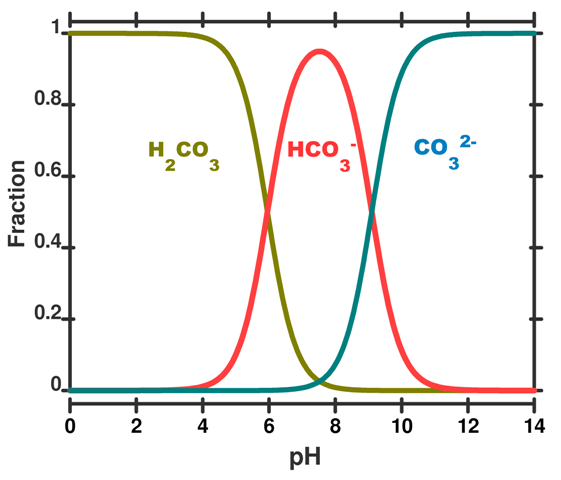Figure 3. A speciation diagram for the carbonic acid system in seawater as a function of pH. The y-axis gives the fraction of each species present. A vertical line drawn at any pH value gives the relative proportion of each species. This plot is simplified to illustrate the concept; in real seawater several other factors like salinity, temperature and pressure are important.

We can run through some example calculations. Concentrations are given as moles per kg of seawater. For prior to the industrial revolution we have taken a pH of 8.25. Other input parameters are a total dissolved inorganic carbon concentration of 2100 x10-6 mol kg-1 (total dissolved inorganic carbon is the sum of the species described in post 5, temperature = 15 deg C, and the salinity = 35. Under these conditions, which are typical for the ocean, the concentration of H2CO3, HCO3 and CO32– would have been 10 x10-6 mol kg-1­, 1830 x10-6 mol kg-1­ and 260 x10-6 mol kg-1  of seawater, respectively (Roy constants used - see below).

The calculated values add to give the total dissolved inorganic carbon of  2100 x10-6 mol kg-1 and HCO3 and CO32– are roughly in a 7:1 ratio (the carbonic acid does not really contribute, because, as expected it is 170 times less than the HCO3). Today, a after a decrease in pH to 8.14 – and an increase of 29% in the concentration of H3O+ – typical calculated concentrations of HCO3 and CO32– are about 1880 x10-6 mol kg-1­ and 210 ×10-6 mol kg-1, respectively. You can see that HCO3 and CO32– are now roughly in 9:1 ratio. The concentration of carbonate CO32– has changed by –25% ((260-210)/210 × 100), but the concentration of bicarbonate HCO3 has only changed by 3% ((1880-1830)/1830) × 100.

We have made several simplifications here as the calculations are complex and beyond the scope of a blog post. Indeed, the full calculations are not encountered before postgraduate study. However, if you would like to try it yourself, you can download a program, written by one of us (KH) called SWCO2. It is available at the University of Otago. You will need to take some care – actual realistic values for the various entry parameters fall within a relatively narrow range.

If you do have a go yourself you will discover yet another layer of complexity: There are several sets of equilibrium constant, K, values to choose from. The reasons are complex and rest on the way that these values are determined experimentally and, though each set of values is internally consistent, each set uses a slightly different set of initial assumptions.

Now, just to further complicate things, we will introduce another equation:K for this reaction is about 10-3. That is, the ratio of left to right is about 1,000:1. This means that, to a first approximation, seawater (dominated by HCO3), has only a little bit of CO2 and CO32-. More importantly, it also shows that if we add CO2 to seawater, CO2 will spontaneously react with CO32– to form 2HCO3 because K for the reverse reaction is 103. In a later post we put some numbers to this concept.

Ocean acidification is caused by absorbance of atmospheric CO2 by seawater. We now see that this acidification of surface seawater is causing the removal of CO32–: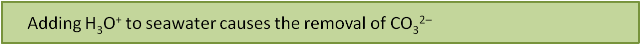But why should this be a problem? We know from Equation 1 that calcification uses bicarbonate HCO3, not carbonate CO32-. Why does it matter if some carbonate from the oceans is converted to bicarbonate? Won't that actually help the shellfish? We explain why not, and just where the acid is coming from, over the next few posts.

Written by Doug Mackie, Christina McGraw , and Keith Hunter . This post is number 8 in a series about ocean acidification. Other posts: Introduction , 1, 2, 3, 4, 5, 6, 7, 8, 9, 10, 11, 12, 13, 14, 15, 16, 17, 18, Summary 1 of 2, Summary 2 of 2.1. Thanks again for the series. It's been 20 years since I studied any chemistry (and only got up to introductory undergraduate level as part of my engineering degree) so it's a good refresher for the things I knew, and educational about the things I didn't. While I find the wait for the next 'episode' somewhat frustrating, I think the pace of the articles is good - laying the foundations firmly along the way.
2. Thank you Bern. We knew if we chucked down 15,000 words in one go people would not read any of it. We are taking in the comments as we go and will incorporate answers into the booklet that comes out at the end.
3. I appreciate all the effort Doug Mackie has made to produce this series, but I find this posting confusing. Eq. 12 by itself doesn't really imply that adding CO2 decreases CO3--, since the total concentration of carbon would be larger. Consider the opposite: removing all CO2. The result would not be more CO3--, since there wouldn't be any carbon left. Figure 3 is misleading because it seems to imply some agent other than CO2 is changing the pH.
4. None of the equations by themselves tell us anything. Our point is that we need to consider all these equations together. In several of the later posts we show how we can use the K and Q values that we have introduced here to know the extent to which each equation occurs and how to work out what the overall outcome is. Read what we say again:
K for [equation 12] is about 10-3. That is, the ratio of left to right is about 1,000:1. This means that, to a first approximation, seawater (dominated by HCO3-), has only a little bit of CO2 and CO32-. More importantly, it also shows that if we add CO2 to seawater, CO2 will spontaneously react with CO32- to form 2 HCO3- because K for the reverse reaction is 103.
That is if you reverse the reaction then it still favours, with a 1000:1 ratio the side with HCO3- over the side with CO2. We are unclear how you draw your conclusion about figure 3.
5. Jeff T: Figure three shows the distribution of carbon in the three forms when the pH is at different levels no matter what makes the pH change. If you put carbonate and/or bicarbonate in a beaker and then add nitric acid the proportions of each form of carbonate will change. The proportions will also change if you add any other acid, hydrochloric acid, acetic acid (vinegar), or carbonic acid. If you use CO2 to lower the pH then the fractions behave just like the figure shows, even as the total amount of carbon increases. You can also increase the pH by adding any kind of base, for example sodium hydroxide. And you can increase the pH by adding carbonate (CO332-). In that case the total amount of carbon also increases while the fractional distribution shifts to the right on the graph. Removing CO2 decreases the pH (by eq 8 it removes H3O+) and so the fractional distribution again shift right, even though this time the total amount of carbon decreases.. Figure 3 is very powerful precisely because it separates the total amount of carbon from the fraction in each form.
6. Thank you Sarah. We did think about a more complex figure with multiple axes that included changing total carbon but we felt it would not have been easily understood.
7. Christina, I guess my difficulty with the post arises from the fact that it includes only three equations (8, 9 and total carbon content = sum of carbon species) to determine four parameters (concentrations of H3O+, CO2, HCO3- and CO3--). Since Eq. 12 is the difference between Eq. 8 and 9, it doesn't provide a fourth independent relation. I suppose that you don't give a fourth equation because there are really many relations and species concentrations required. As I said, I appreciate the effort, but I don't think you've solved the problem of presenting the issue clearly. Sarah, the example computation following Figure 3 assumes that total carbon content is constant. That's why it shows a decrease in concentration of CO3--. Wouldn't an example that decreases pH by adding total carbon show an increase in CO3-- concentration, even though the ratio of CO3-- concentration to other carbon species would decrease. It may be that the ratio is more important than the concentration, but the post doesn't say so. It would be informative to remake Figure 3 to show the fractions (or concentrations, whichever is more important) of the three carbon species as a function of the total CO2 added to pre-industrial seawater.
8. Jeff, Are you the Jeff T who supports Piers Corbyn? If so, it would explain a lot. If not then consider what you have said again about the number of components and equations. What happens if you have 4 doors to open with 4 keys but the keys are mixed up? Once you have correctly identified 3 of the keys do you need to test the 4th key to identify its door? The figure shows that the components are not independent. The equations link them. All aspects of the CO2 system can be described by any two of the composition parameters. e.g. CT and pH are sufficient to determine AT, pH and CO2. Your inability to understand the figure does not appear to be widely shared. By all means prepare a figure yourself and post it here.
9. Jeff, Figure 3 could be made with the Y-axis in terms of concentration instead of fraction and it would look the same. For example, instead of 1.0 it the value could be 2100 x10-6 mol kg-6. Adding more carbon to the system would make all three lines increase together. So, if the new concentration is 10% higher (it isn't in the real world!) all three lines would be 10% higher on that scale but their proportions would stay the same. Another way to think about this is to consider the CO3-2 line at around pH 8.1. If you add a significant amount of CO2 then all three lines go up when the Y-axis is in concentration units. But, by eqn 12, adding CO2 causes some of the CO3-2 to convert to HCO3- and the new distribution defines a new more acidic pH. Actually, the 2% increase in ocean carbon would only shift the concentration lines up (together) by a tiny amount (2%). But because the system at pH 8.1-8.2 is far down on the slippery slope of low carbonate, it makes a big change in percent CO3-2. Your suggestion that the ratio is more important than the concentration for defining the pH is correct. The complication is that in this system carbon is both the main thing controlling the pH (by the ratio of bicarbonate/carbonate) and the thing making the pH shift (because CO2) in water make carbonic acid, which reacts with carbonate to change the ratio, eqn 12). Neither the figure nor the post are "using only three equations to define four parameters". They are using the equations to understand the behavior of the system as a function of pH (H3O+). If you want to calculate the pH for a system containing these carbon species, then the equation you need is: 2H2O --> H3O+ + OH-. pKa=14.
10. Doug, please read comment 8 (14:44 PM) carefully. I'm a different Jeff. I studied what you wrote; I'd like to understand it; but I don't. Generally, one needs four equations to solve for four coupled parameters. Sarah and I are the only non-authors to make substantive comments. Do you really conclude from such a small sample that everyone else understood the post? I don't know what CT and AT are. Sarah, if the species ratios are more important than concentrations (for the health of corals or clams, say), it would help a lot for the post to explain that. The equation for water at the end of comment 9 (15:02 PM) isn't sufficient; water plus CO2 doesn't produce pH=8.
11. @Keith Hunter Thanks for the free CO2Calc application. @Jeff T When you increase CO2 concentration in the atmosphere the equilibriums 7, 8, and 9 start shifting and the result is a lower pH, more HCO3(-) and less CO3(2-). In my opinion figure 3 shows this perfectly. Even though you increase the amount of carbon in the oceans by increasing the CO2, you get less CO3(2-). Less CO3(2-) means that equilibrium CaCO3 = Ca(2+) + CO3(2-) shifts to the right and the calcifying organisms won't like that. Look at the table in The Encyclopedia of Earth where you can see some estimates about these concentrations for different dates. And I think they mean by CT and AT : total dissolved CO2 and total alkalinity.
12. JeffT, the algebra gets a wee bit tricky but it is easy to define the fraction of each species only in terms of K1, K2 and [H3O+]. Try googling dissociation of a diprotic acid. If there is huge interest we may consider an extra post to go through the equations.
13. Jeff, You are correct that a mixture of only CO2 and water won't make pH 8 (I did not mean to suggest that). Pure water in equilibrium with air is about pH 5.7. Weathering has added CaCO3 to the oceans over millions of years, which dissolves to the base CO32-. Alkalinity (AT) is a measure of how much "excess" base is in the system, for example, how much CO32- came in with Ca2+ instead of with H+ as the counter ion (to balance the charges, you can't add negative charge without adding + charges too). The charge balance in the system provides another constraining equation. The sum of charges of all the species present must add up to zero. The pH is determined by how much of the charge ends up being H3O+ after all the equilibrium equations are satisfied.
14. Thanks Sarah, though overall weathering actually produces mainly bicarbonate HCO3- (Eq. 4) We discuss alkalinity in post 12. Seawater alkalinity is a more complex expression than that for freshwater and also includes species like B(OH)4-, SiO(OH)3-, and HF.
15. Doug, Correct. I'm was trying to simplify to the crux of Jeff's concern, which seems to be CT plus water. Additional contributions to alkalinity are critical for real world calculations, but the basic (and acidic!) chemistry ideas can be understood with a simplified mixture.
16. #second summary post